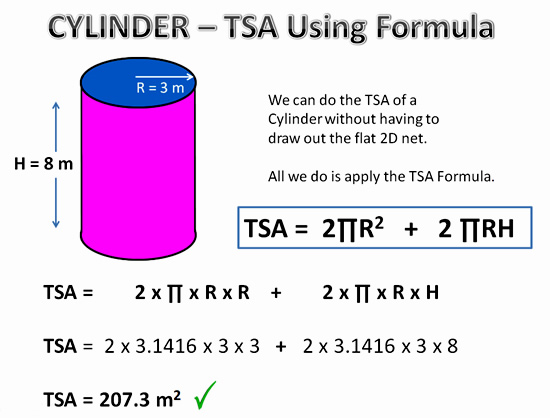# Java Program Calculate Total Surface Area Of Cylinder | 3 Ways

How to write a java program to calculate the total surface area of a cylinder? Well! That’s quite simple here we share in three different ways to write Java code for the total surface area of a cylinder. Soon online execution tool is going to be embedded for each program such that you can execute here itself. In case if you need to know any more information about the following program, do comment here at the end of the post. Check out the table of contents.

[table id=2 /]

Q: How to calculate the total surface area of a cylinder?

A: The total surface area of the cylinder includes a area of the circular top + curved surface area.

## Here is the code in three different ways to calculate TSA

The formula to calculate the total surface area of the cylinder in math?Image source: http://passyworldofmathematics.com/

### Java code: Example – 1 ( Standard values )

Here we use simple standard values by using the above formula. For any mathematical oriented Java program, you can use the following format to execute. Below we do cover in more than two methods with outputs and online execution tool.

Output :

#### Java Code For TSA: 2 ( Command Line Arguments )

Here we use command line arguments. If you have no idea about java command line arguments, then do check out the guide. Command line arguments, where inputs displayed on the output console itself. Check out how the output will show alike.

Output: Using command line arguments ( Example Code – 2 )

##### Java Code TSA: 3 ( Return Invoke Method )

( Updated )We got a mail from one user asked, is there any way on how to write a java program other than using static and command line arguments? Here we go another method using return to invoke a method. We do implement this on all our basic formula oriented programs. Do check it out 🙂

Output For Java Code: 3
That’s all three simple ways to find the total surface area of a cylinder. In case if you need any more information then do check out the beginner programs here. Online execution tool is soon going to be implemented on our site where you can run each program individually.

Check Out More Tutorials:

x

## X Star Pattern Java Program – Patterns

Java program to print X star pattern program – We have written the below print/draw ...